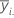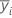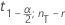# Methods and formulas for the confidence intervals in One-Way ANOVA

Select the method or formula of your choice.

## Mean

### Formula

The average of the observations at a given factor level.

### Notation

TermDescription
ni number of observations at factor level i
yij value of the j th observation at the i th factor level

## Standard deviation (StDev)

### Notation

TermDescription
yij observations at the i th factor levelmean of observations at the i th factor level
ni number of observations at the i th factor level

## Pooled standard deviation

### Formula

The common variance for all observations. The pooled variance is:
The pooled standard deviation is the square root of the above formula. An equivalent form follows:

The pooled standard deviation is equivalent to S, which is in the output and is equal to:

### Notation

TermDescription
yij j th observation of the response for the i th factor levelsample mean for factor level i
ni number of observations for the ith factor level
nT total number of observations
si 2 variance of observations
r number of levels of the factor
MSMean Square

## Individual confidence intervals

### Formula

The confidence intervals are calculated for each factor level mean using the pooled standard deviation. The formula is:

### Notation

TermDescription
nT total number of observations
r number of factor levels
S pooled standard deviationinverse cumulative distribution function from the t distribution at 1− α/2 with nTr degrees of freedom
By using this site you agree to the use of cookies for analytics and personalized content.  Read our policy